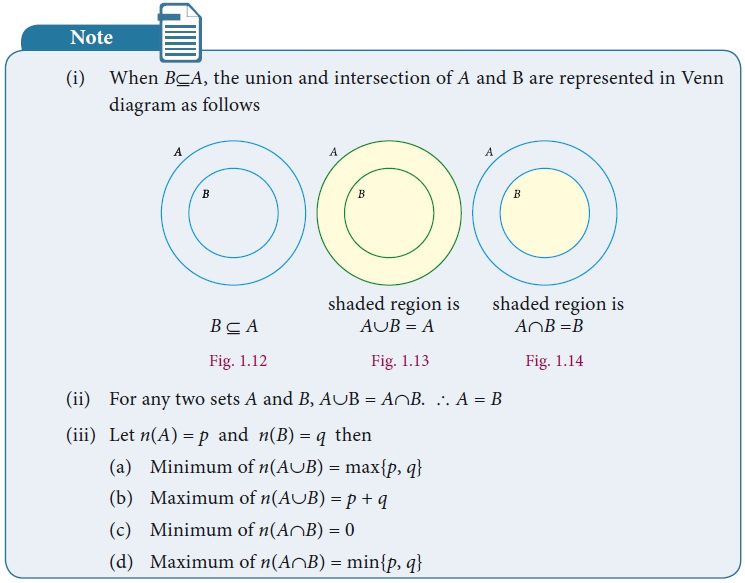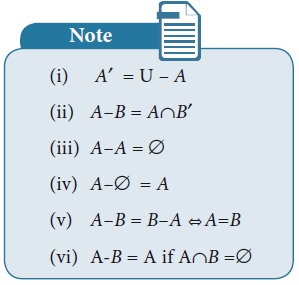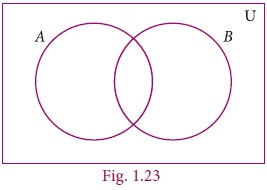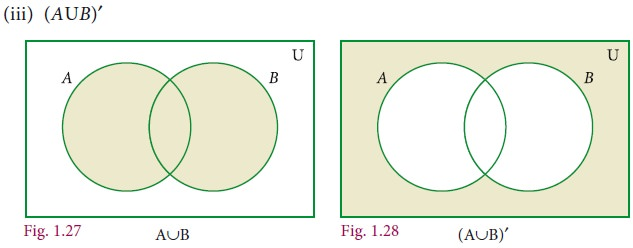Home | Set Operations

# Set Operations

We started with numbers and very soon we learned arithmetical operations on them.

Set Operations

We started with numbers and very soon we learned arithmetical operations on them. In algebra we learnt expressions and soon started adding and multiplying them as well, writing (x2+2) (x-3) etc. Now that we know sets, the natural question is, what can we do with sets, what are natural operations on them ?

What can we do with sets ? We can pick an element. But then which element ? There are many in general, and hence “picking an element” is not an operation on a set. But like we did with addition, subtraction etc, we can try and think of operations that combine two given sets to get a new set. How can we do this ?

A simple way is to put the two sets together. This gives us a new set, and exactly one set, so it is an operation. We could pick out exactly the common elements of the two given sets. That’s an interesting operation too. We could talk of all the elements not in the given set. But this is problematic: we can list the elements in a set, but which are the elements not in it ? Almost anything. We know that 5734 is in the set of natural numbers, but we also know that my chair is not in it, an elephant is not in it, and so on. How could we ever hope to describe all these elements ? But then when we speak of sets of numbers we are clearly not talking of elephants ! So we should really speak of numbers not in the set of natural numbers. For instance we could implicitly fix the integers and talk of integers not in the set of natural numbers. In general, we call this “fixed” set the universal set (relative to which we speak of what is or not in a given set).

When two or more sets combine together to form one set under the given conditions, then operations on sets can be carried out. We can visualize the relationship between sets and set operations using Venn diagram.

## 1. Universal Set

A Universal set is a set which contains all the elements of all the sets under consideration and is usually denoted by U.

For example,

i. If we discuss about elements in Natural numbers, then the universal set U is the set of all Natural numbers. U={x : x N}.

ii. If A={earth, mars, jupiter}, then the universal set U is the planets of solar system.

## 2. Complement of a Set

The Complement of a set A is the set of all elements of U (the universal set) that are not in A.

It is denoted by A or Ac. In symbols A= {x : xU, xA}

### Venn diagram for complement of a setFor example,

If U = {all boys in a class} and A= {boys who play Cricket}, then complement of the set A is A= {boys who do not play Cricket}.

### Example 1.9

If U = {c, d, e, f, g, h, i, j} and A = { c, d, g, j} , find A.

Solution

U = {c, d, e, f, g, h, i, j}, A = {c, d, g, j}

A ={e, f, h, i}## 3. Union of Two Sets

The union of two sets A and B is the set of all elements which are either in A or in B or in both. It is denoted by AB and read as A union B.

In symbol, AB = {x : x A or xB}

### The union of two sets can be represented by Venn diagram as given belowFor example,

If P ={Asia,Africa, Antarctica, Australia} and Q = {Europe, North America, South America}, then the union set of P and Q is PQ = {Asia, Africa, Antartica, Australia, Europe, North America, South America}.### Example 1.10

If A={1, 2, 6} and B={2, 3, 4} , find AB.

Solution

Given A={1, 2, 6}, B={2, 3, 4}

AB={1, 2, 3, 4,6}.

### Example 1.11

If P={m, n} and Q= {m, i, j}, represent P and Q in Venn diagram and find PQ.

Solution

Given P={m, n} and Q= {m, i, j}

From the diagram,

PQ={n, m, i, j}.## 4. Intersection of TwoSets

The intersection of two sets A and B is the set of all elements common to both A and B. It is denoted by AB and read as A intersection B.

In symbol , AB={x : xA and xB}

### Intersection of two sets can be represented by a Venn diagram as given belowFor example,

If A = {1, 2, 6}; B = {2, 3, 4}, then AB = {2} because 2 is common element of the sets A and B.Can we determine n(A B) in terms of n(A) and n(B)? This seems to be difficult, but what about n(A B) in terms of n(A) and n(B) ?

Notice that all the elements in A are in (A B), and all the elements in B also in A B

Can we say that n(A B) = n(A) + n(B) ?

Unfortunately not. Consider an element is common to both A and B? Of course it is in A union B, but is counted both in A and in B and we don’t want to count the same element twice in A union B !

So indeed, n(A B) = n(A) + n(B) – n(A B). But then it is easy to see that n(A B) = n(A) + n(B) – n(A B). So between the union and the intersection we need to know one to determine the other, given n(A) and n(B).

### Example 1.12

Let  A = {x : x is an even natural number and 1< x ≤ 12} and

B = { x : x is a multiple of 3, x N and x≤12} be two sets. Find AB.

Solution

Here  A = {2, 4, 6, 8, 10, 12} and B = {3, 6, 9, 12}

AB = {6, 12}

### Example 1.13

If A = {2, 3} and C = { }, find AC.

Solution

There is no common element and hence AC ={ }## 5. Difference of Two Sets

Let A and B be two sets, the difference of sets A and B is the set of all elements which are in A, but not in B. It is denoted by A–B or A\B and read as A difference B.

In symbol, AB = { x : x A and x B}

BA = { y : y B and y A}.

### Venn diagram for set difference### Example 1.14

If A={–3, –2, 1, 4} and B= {0, 1, 2, 4}, find (i) AB (ii) BA.

Solution

AB ={–3, -2, 1, 4} – {0, 1, 2, 4} = { -3, -2}

BA = {0, 1, 2, 4} –{–3, -2, 1, 4} = { 0, 2}## 6. Symmetric Difference of Sets

The symmetric difference of two sets A and B is the set (AB)(BA). It is denoted by AΔB.

A  B={ x : x AB or x BA}

### Example 1.15

If A = {6, 7, 8, 9} and B={8, 10, 12}, find AΔB.

Solution

AB = {6, 7, 9}

BA = {10, 12}

AΔB = (AB)(BA) = {6, 7, 9}{10,12}

AΔB = {6, 7, 9, 10, 12}.

### Example 1.16

Represent AΔB through Venn diagram.

Solution

AΔB= (A–B) (B–A)## 7. Disjoint Sets

Two sets A and B are said to be disjoint if they do not have common elements.

In other words, if AB=, then A and B are said to be disjoint sets.### Example 1.17

Verify whether A={20, 22, 23, 24} and B={25, 30, 40, 45} are disjoint sets.

Solution

A = {20,22, 23, 24} , B={25, 30, 40, 45}

AB = {20,22, 23, 24} {25, 30, 40, 45}

= { }

Since AB = , A and B are disjoint sets.### Example 1.18

From the given Venn diagram, write the elements of

(i) A (ii) B (iii) AB (iv) BA (v) A (vi) B (vii) USolution

(i) A = {a, e, i, o, u}

(ii) B = {b, c, e, o}

(iii) A–B = {a, i, u}

(iv) B–A = {b, c}

(v) A′ = {b, c, d, g}

(vi) B′ = {a, d, g, i, u}

(vii) U = {a, b, c, d, e, g, i, o, u}

### Example 1.19

Draw a Venn diagram similar to one at the side and shade the region representing the following sets(i) A(ii) (AB)   (iii) (AB)

SolutionTags : Solved Example Problems | Mathematics , 9th EM Mathematics : Set Language
Study Material, Lecturing Notes, Assignment, Reference, Wiki description explanation, brief detail
9th EM Mathematics : Set Language : Set Operations | Solved Example Problems | Mathematics